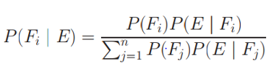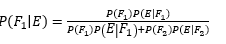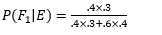# Bayes Theorem

## Bayes Formula

Let S be the Sample space and let F1 ,F2………Fn­    be the n mutually exclusive and exhaustive events associated with the random experiments. If E is any event which occurs with those n mutually exclusive events, thenSome Important points about Bayes Theorem
 Event F1  , F2……Fn They are called hypothesis P(F1) …… P(F2) These probabilities are called priori probabilities as they exist before we obtain any information from experiment P( E/F1) ……. P(E/F­n) These probabilities are called likelihood probabilities as they tell us how event A under consideration occurs P(F1/E) …. P(Fn/E) These probabilities are called the posterior probabilities as they are determined after the result of the experiment is known

## Bayes Formula Solved examples

Examples:

Question
Of all the smokers in a particular district in India   40% prefer brand X   and 60% prefer brand Y. Of those smokers who prefer brand X, 30% are females, and of those who prefer brand Y, 40% are female. What is the probability that a randomly selected smoker prefers brand A, given that the person selected is a female?
Solution
Let F1 = "prefer brand A",
F2 = "prefer brand B" and
E is the event "female".
We need to find out   P(F1|E)
Now from Bayes formulaIn this particular situationSoOr
P(F1|E) =1/3

## Related Topics

Go back to Class 12 Main Page using below links